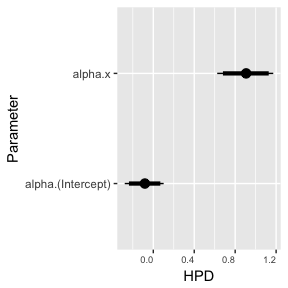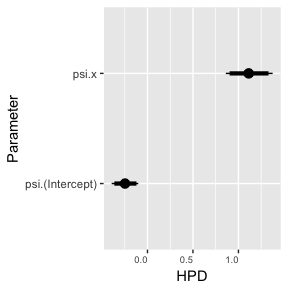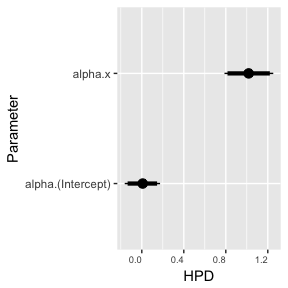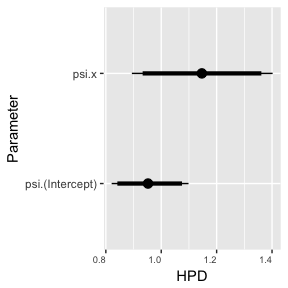# Accounting for Weights in the Model

#### 2018-01-28

In some cases the response variable may be an average of responses from within the same subject or group. In this case, the variances for two observations having the same covariates will not be identical if the size of the groups vary. Instead, it will be inversely proportional to the size of the group. Mathematically, if the observed response is the group average $$\bar{Y}_i=\sum_{j=1}^n_i Y_{ij}/n_i$$ and $$Y_{ij} \sim N(\mu_i,\sigma^2_i)$$ where $$\sigma^2_i$$ may vary by group and depend on covariates then $\bar{Y}_i \sim N(\mu_i,\sigma^2_i/n_i).$ This situation can be accommodated in dalmatian by specifying a column of weights within the data frame that provides the group size. Here is an example with simulated data that demonstrates this feature and shows the importance of properly accounting for weights.

# Simulated data

Data for this example are contained in the data frame weights.df saved within the file weights-data-1.RData. The data were generated from the model $Y_{ij} \sim N(x_i,\exp(1+x_i)^2)$ with $$i=1,\ldots,100$$ indexing the groups and $$j$$ the observarions within groups. In the data, the number of observations per group ranges from 5 to 43.

## Load library
library(dalmatian)

data(weights_1_simulate)
## Warning in data(weights_1_simulate): data set 'weights_1_simulate' not
## found
head(weights.df)
##    n          x          y
## 1 43 -1.0000000 -1.0689833
## 2  9 -0.9797980 -1.0056912
## 3 15 -0.9595960 -0.7625561
## 4 10 -0.9393939 -0.6073663
## 5 13 -0.9191919 -0.7382720
## 6  9 -0.8989899 -0.9372230

The three columns in the data frame record the number of responses per group (n), the value of the covariate (x), and the mean response (y).

# Model 1: No Weights

First we run the model with no weights.

## Mean model
mymean=list(fixed=list(name="alpha",formula=~ x,
priors=list(c("dnorm",0,.001))))

## Variance model
priors=list(c("dnorm",0,.001))))

## Set working directory
## By default uses a system temp directory. You probably want to change this.
workingDir <- tempdir()

## Define list of arguments for jags.model()

## Define list of arguments for coda.samples()
cs.args = list(n.iter=1000)

## Run the model using dalmatian
results1 <- dalmatian(
df = weights.df,
mean.model = mymean,
variance.model = myvar,
jags.model.args = jm.args,
coda.samples.args = cs.args,
response = "y",
overwrite = TRUE,
debug = FALSE
)
## Step 1: Generating JAGS code...Done
## Step 2: Generating JAGS data...Done
##     Computing singular value decompositions to improve mixing...Done
## Step 3: Generating initial values...
##     Running three parallel chains by default...Done
## Step 4: Running model in JAGS
##     Initializing model
## Compiling model graph
##    Resolving undeclared variables
##    Allocating nodes
## Graph information:
##    Observed stochastic nodes: 100
##    Unobserved stochastic nodes: 4
##    Total graph size: 1213
##
## Initializing model
##
##    Generating samples
## Done
## Step 5: Tidying Output...Done
## Numerical summary statistics
summary(results1)
##
## Iterations = 1001:2000
## Thinning interval = 1
## Number of chains = 3
## Sample size per chain = 1000
##
## Posterior Summary Statistics for Each Model Component
##
## Mean Model: Fixed Effects
##              Mean Median   SD Lower 95% Lower 50% Upper 50% Upper 95%
## (Intercept) -0.08  -0.08 0.10     -0.28     -0.14     -0.01      0.10
## x            0.90   0.91 0.14      0.63      0.81      0.99      1.17
##
## Variance Model: Fixed Effects
##              Mean Median   SD Lower 95% Lower 50% Upper 50% Upper 95%
## (Intercept) -0.25  -0.25 0.07     -0.39     -0.30     -0.20     -0.10
## x            1.12   1.11 0.13      0.87      1.02      1.19      1.38
## Graphical summaries
caterpillar1 <- caterpillar(results1)From the summaries we can see that that the intercept in the variance model is being underestimated. The true value is 1, but the posterior mean is -.24 with 95% HPD interval (-.39,-.12).

# Model 2: Weights

We now re-run the model including the weights.

## Specify column containing weights
myvar\$weights = "n"

## Run model

results2 = dalmatian(df=weights.df,
mean.model=mymean,
variance.model=myvar,
jags.model.args=jm.args,
coda.samples.args=cs.args,
response="y",
overwrite = TRUE,
debug=FALSE)
## Step 1: Generating JAGS code...Done
## Step 2: Generating JAGS data...Done
##     Computing singular value decompositions to improve mixing...Done
## Step 3: Generating initial values...
##     Running three parallel chains by default...Done
## Step 4: Running model in JAGS
##     Initializing model
## Compiling model graph
##    Resolving undeclared variables
##    Allocating nodes
## Graph information:
##    Observed stochastic nodes: 100
##    Unobserved stochastic nodes: 4
##    Total graph size: 1312
##
## Initializing model
##
##    Generating samples
## Done
## Step 5: Tidying Output...Done
## Numerical summary statistics
summary(results2)
##
## Iterations = 1001:2000
## Thinning interval = 1
## Number of chains = 3
## Sample size per chain = 1000
##
## Posterior Summary Statistics for Each Model Component
##
## Mean Model: Fixed Effects
##             Mean Median   SD Lower 95% Lower 50% Upper 50% Upper 95%
## (Intercept) 0.01   0.01 0.09     -0.16     -0.04      0.08      0.18
## x           1.02   1.02 0.12      0.79      0.94      1.10      1.25
##
## Variance Model: Fixed Effects
##             Mean Median   SD Lower 95% Lower 50% Upper 50% Upper 95%
## (Intercept) 0.95   0.95 0.07      0.82      0.91      1.01      1.09
## x           1.15   1.15 0.13      0.88      1.05      1.22      1.39
## Graphical summaries
caterpillar2 <- caterpillar(results2)The new output shows that the estimate of the intercept for the variance model, .95, is now very close to the truth and the 95% credible interval, (.82,1.10) easily covers the true value of 1.